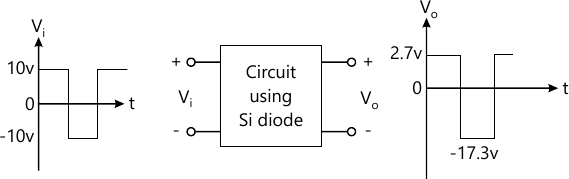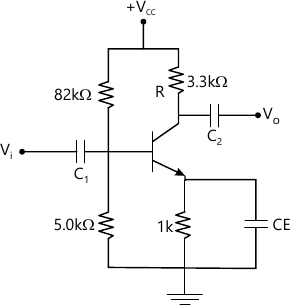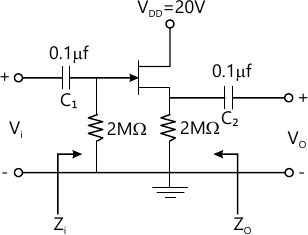MORE IN Analog Electronics
Total marks: --
Total time: --
INSTRUCTIONS
(1) Assume appropriate data and state your reasons
(2) Marks are given to the right of every question
(3) Draw neat diagrams wherever necessary

1 (a) State and explain the various resistance levels of the semiconductor diode.
6 M
1 (b) Explain the working of a full wave centre tapped rectifier. Also determine ripple factor, efficiency and voltage regulation.
10 M
1 (c) Design a suitable circuit represented by the box shown below, which has the input and output waveforms as indicated.4 M

2 (a) Name different biasing methods of transistor. With circuit diagram analyse the fixed bias circuit, with effect of variation in IB, RC and VCC on Q, point of the load line.
10 M
2 (b) Explain the circuit of transistor switch being used as an inverter.
4 M
2 (c) In a voltage divider bias circuit of BJT. VCC=20 V, RC=10 KΩ, RE=1.5 kΩ, R1=40 kΩ, R2=4 kΩ. Assume silicon transistor β=150. Find IC, VCE and IC(sat) using exact analysis.
6 M

3 (a) Define h-parameters and hence derive h-parameters model of CE-BIT.
6 M
3 (b) Explain with a neat circuit diagram of emitter configuration. Justify how voltage gain is nearly equal to one.
6 M
3 (c) For the circuit shown below determine VCC, if AV=160 and r0=100 kΩ. Take β=100.8 M

4 (a) Draw the single stage RC coupled BJT amplifier and discuss the effect of (low frequency response): i) Input capacitance Cb ii) output capacitance CC and iii) Emitter by pass capacitance Ce on frequency response.
5 M
4 (b) Prove that miller effect of input capacitance CMi=(1-Av) Cf and output capacitance $C_{M0} = \left ( 1- \dfrac {1} {A_v} \right ) C_f.$
10 M
4 (c) It is desired that the voltage gain of an RC - coupled amplifier at 60Hz should not decrease by more than 10% from its mid bond value. Calculate:
i) the lower 3 dB frequency
ii) the required C if R=2000 Ω.
5 M

5 (a) Derive expression for Zi and Ai for a Darlington emitter follower circuit.
10 M
5 (b) Mention the types of feedback connections. Draw their block diagrams indicating input and output signal.
6 M
5 (c) List the general characteristics of a negative feedback amplifier and write its advantages.
4 M

6 (a) With a neat circuit diagram, explain the operation of a transformer coupled class A power amplifier.
7 M
6 (b) Explain the operation of class B push-pull amplifier and derive its conversion efficiency.
8 M
6 (c) The following distortion reading are available for a power amplifier:
D2=0.2, D3=0.02, D4=0.06 with R1 =3.3A and RC=4Ω Calculate:
i) the THD
ii) the fundamental power component
iii) the total power.
5 M

7 (a) Explain the working of Wien bridge oscillator.
7 M
7 (b) With a neat circuit diagram, explain the operation of BJT Colpitt's oscillator.
6 M
7 (c) A crystal has the following parameter L=0.334 H, CM=1 pF, C=0.065 and R=5.5 kΩ. Calculate the series resonant frequency, parallel resonant frequency and find Q of the crystal.
7 M

8 (a) Draw the JFET common drain configuration (source - follower) circuit. Derive Zi, Z0 and Av using small signal model. Write its characteristics.
10 M
8 (b) Compare JFET and MOSFET.
3 M
8 (c) For the JFET common drain configuration shown below. Given IDSS=10 mA, Vp=-5V, rd=40 kΩ, VGSQ=-2.85V i) calculate Zi and Zo ii) Calculate Av iii) find V0 if Vi=20 mV (p-p).7 M

More question papers from Analog Electronics